# 9 DIFFERENTIAL EQUATIONS DIFFERENTIAL EQUATIONS Unfortunately its impossible

• Slides: 759 DIFFERENTIAL EQUATIONS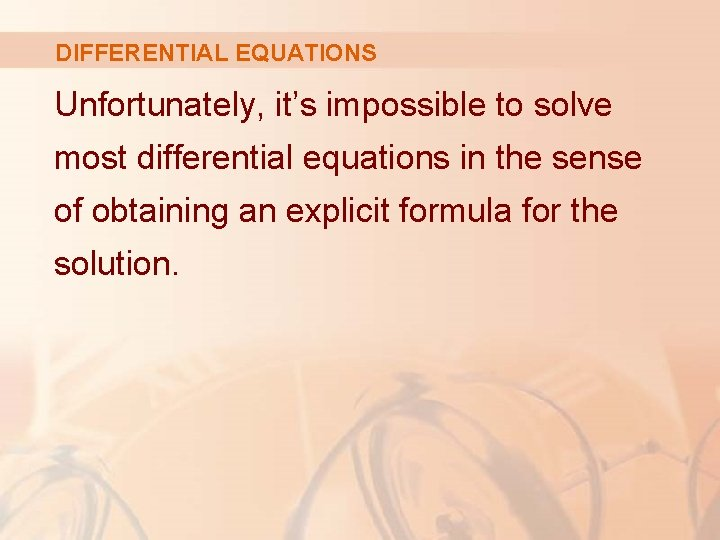DIFFERENTIAL EQUATIONS Unfortunately, it’s impossible to solve most differential equations in the sense of obtaining an explicit formula for the solution.DIFFERENTIAL EQUATIONS Despite the absence of an explicit solution, we can still learn a lot about the solution through either: § A graphical approach (direction fields) § A numerical approach (Euler’s method)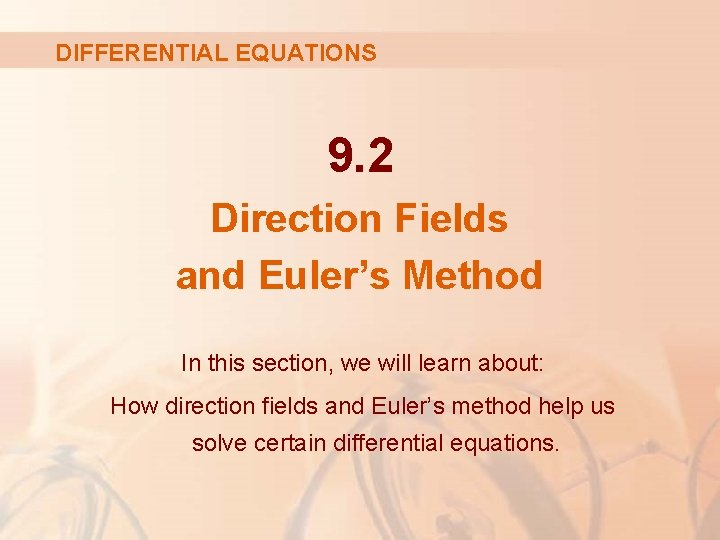DIFFERENTIAL EQUATIONS 9. 2 Direction Fields and Euler’s Method In this section, we will learn about: How direction fields and Euler’s method help us solve certain differential equations.INTRODUCTION Suppose we are asked to sketch the graph of the solution of the initial-value problem y’ = x + y y(0) = 1 § We don’t know a formula for the solution. § So, how can we possibly sketch its graph?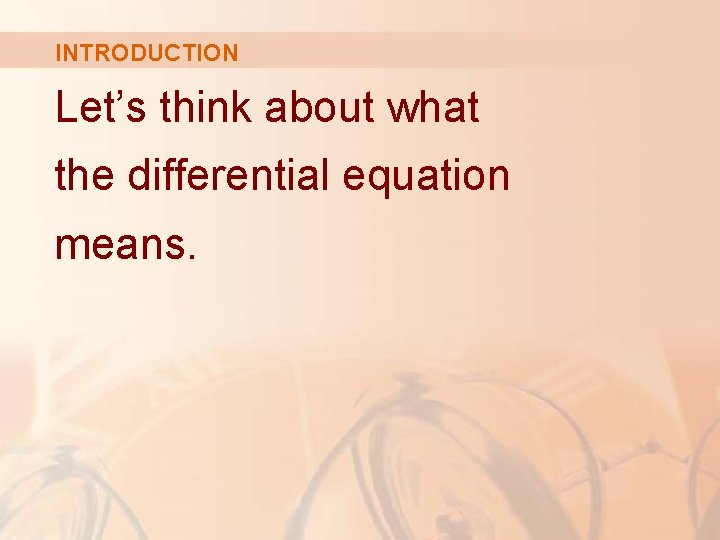INTRODUCTION Let’s think about what the differential equation means.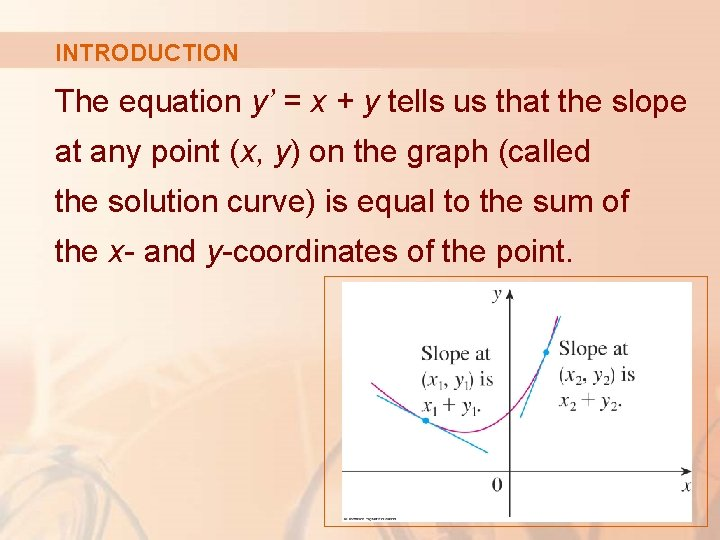INTRODUCTION The equation y’ = x + y tells us that the slope at any point (x, y) on the graph (called the solution curve) is equal to the sum of the x- and y-coordinates of the point.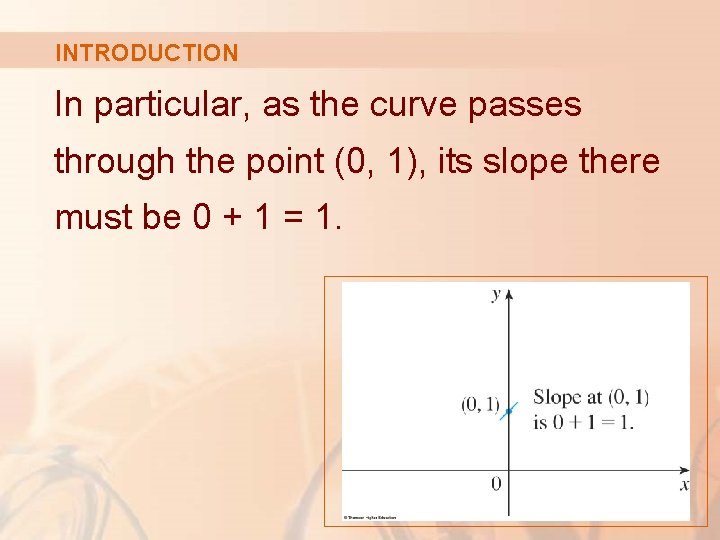INTRODUCTION In particular, as the curve passes through the point (0, 1), its slope there must be 0 + 1 = 1.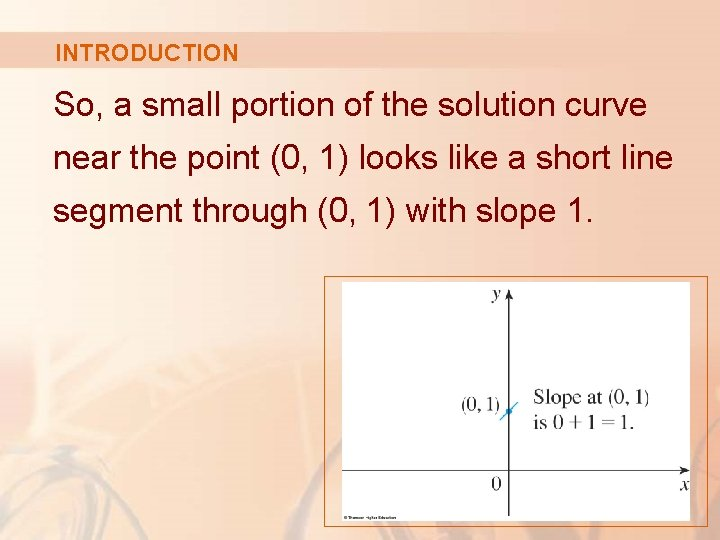INTRODUCTION So, a small portion of the solution curve near the point (0, 1) looks like a short line segment through (0, 1) with slope 1.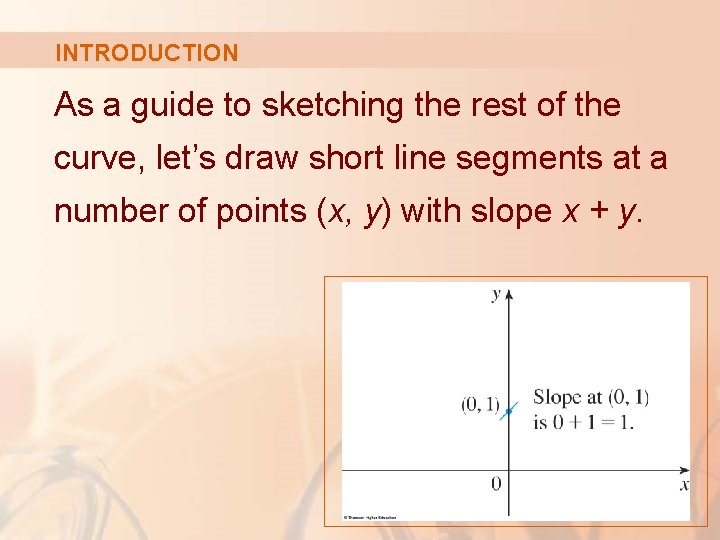INTRODUCTION As a guide to sketching the rest of the curve, let’s draw short line segments at a number of points (x, y) with slope x + y.DIRECTION FIELD The result is called a direction field. § For instance, the line segment at the point (1, 2) has slope 1 + 2 = 3.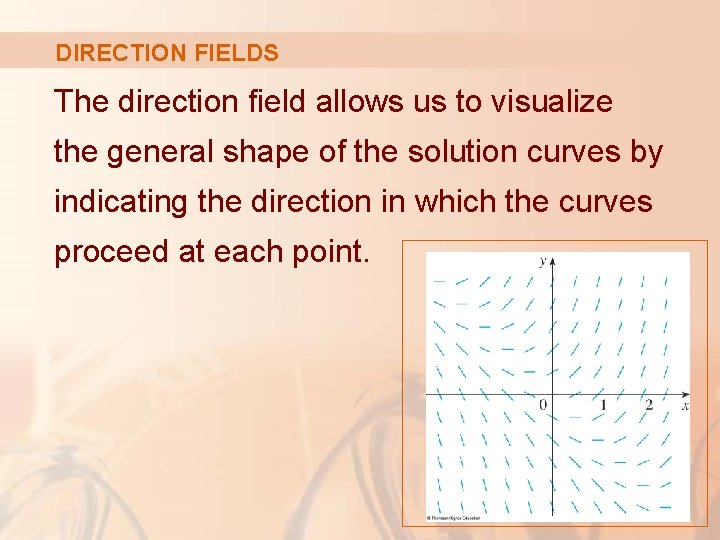DIRECTION FIELDS The direction field allows us to visualize the general shape of the solution curves by indicating the direction in which the curves proceed at each point.DIRECTION FIELDS Now, we can sketch the solution curve through the point (0, 1) by following the direction field as in this figure. § Notice that we have drawn the curve so that it is parallel to nearby line segments.DIRECTION FIELDS In general, suppose we have a first-order differential equation of the form y’ = F(x, y) where F(x, y) is some expression in x and y. § The differential equation says that the slope of a solution curve at a point (x, y) on the curve is F(x, y).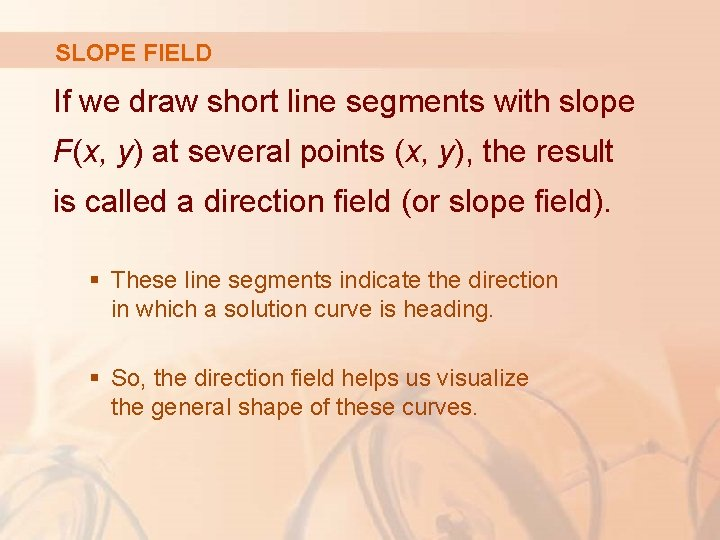SLOPE FIELD If we draw short line segments with slope F(x, y) at several points (x, y), the result is called a direction field (or slope field). § These line segments indicate the direction in which a solution curve is heading. § So, the direction field helps us visualize the general shape of these curves.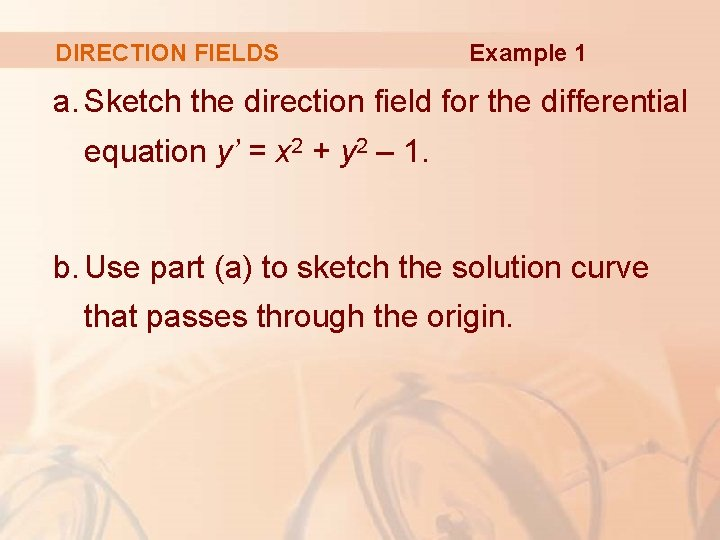DIRECTION FIELDS Example 1 a. Sketch the direction field for the differential equation y’ = x 2 + y 2 – 1. b. Use part (a) to sketch the solution curve that passes through the origin.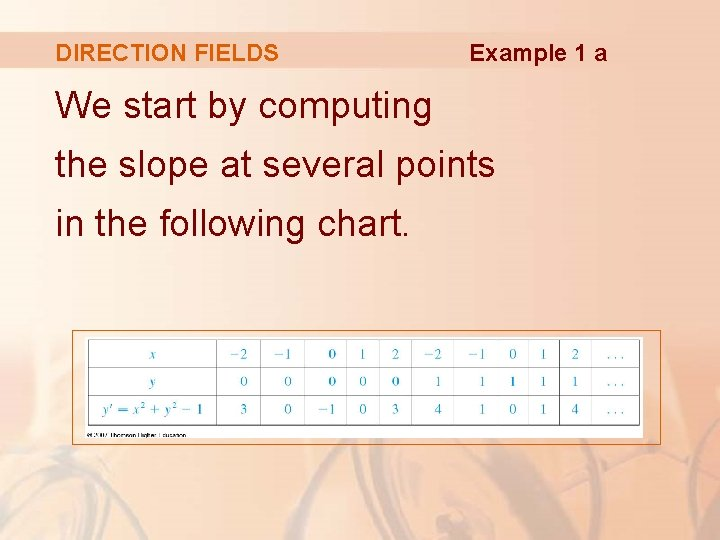DIRECTION FIELDS Example 1 a We start by computing the slope at several points in the following chart.DIRECTION FIELDS Example 1 a Then, we draw short line segments with these slopes at these points.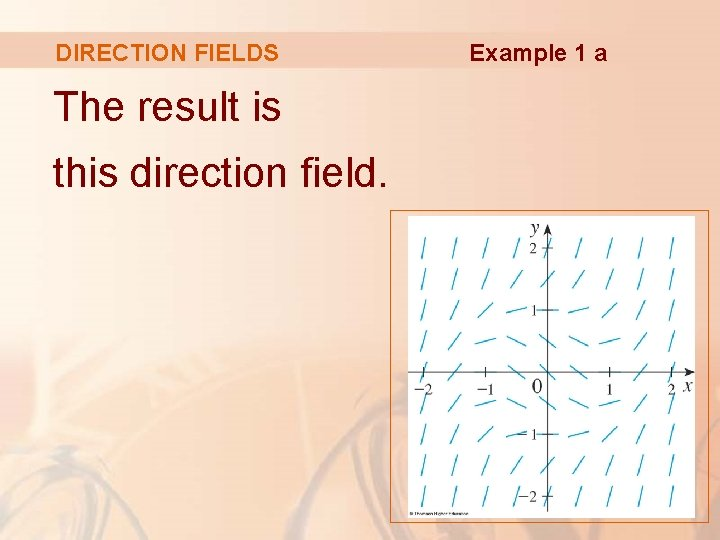DIRECTION FIELDS The result is this direction field. Example 1 a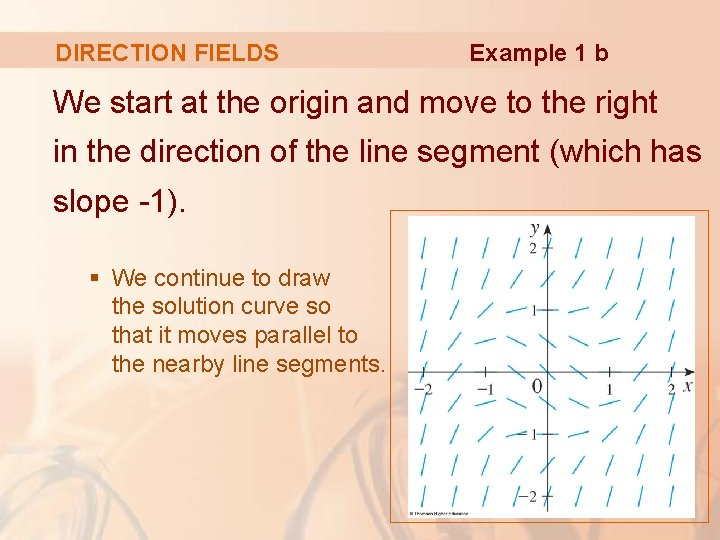DIRECTION FIELDS Example 1 b We start at the origin and move to the right in the direction of the line segment (which has slope -1). § We continue to draw the solution curve so that it moves parallel to the nearby line segments.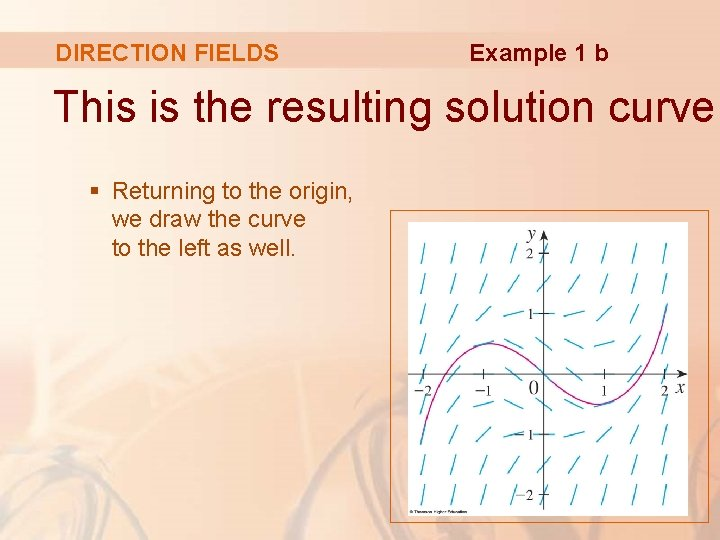DIRECTION FIELDS Example 1 b This is the resulting solution curve. § Returning to the origin, we draw the curve to the left as well.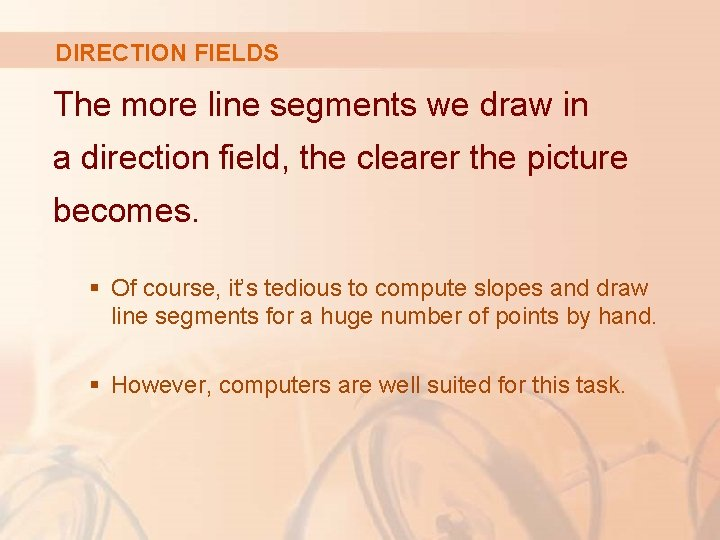DIRECTION FIELDS The more line segments we draw in a direction field, the clearer the picture becomes. § Of course, it’s tedious to compute slopes and draw line segments for a huge number of points by hand. § However, computers are well suited for this task.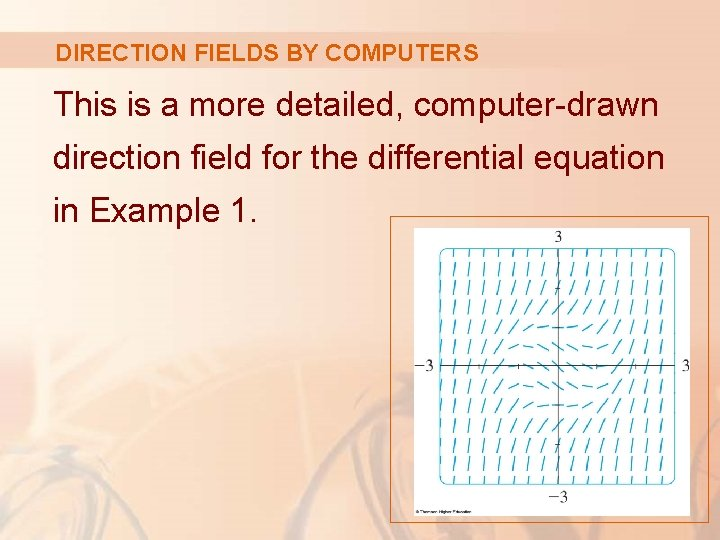DIRECTION FIELDS BY COMPUTERS This is a more detailed, computer-drawn direction field for the differential equation in Example 1.DIRECTION FIELDS BY COMPUTERS It enables us to draw, with reasonable accuracy, the solution curves shown here with y-intercepts -2, -1, 0, 1, and 2.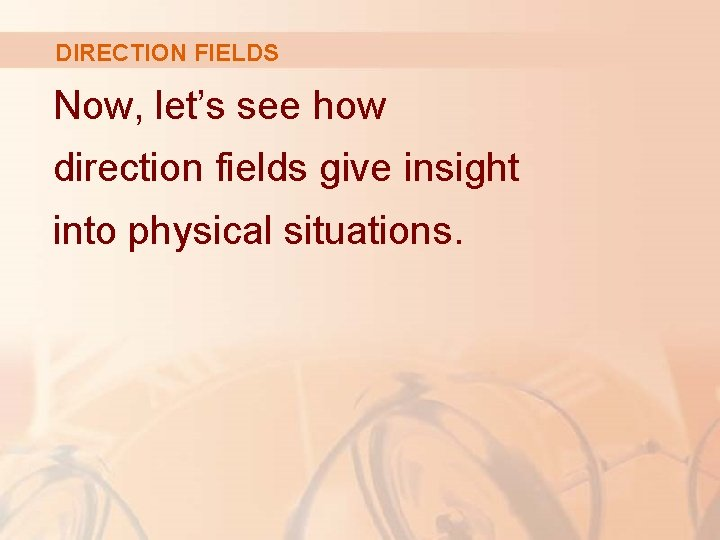DIRECTION FIELDS Now, let’s see how direction fields give insight into physical situations.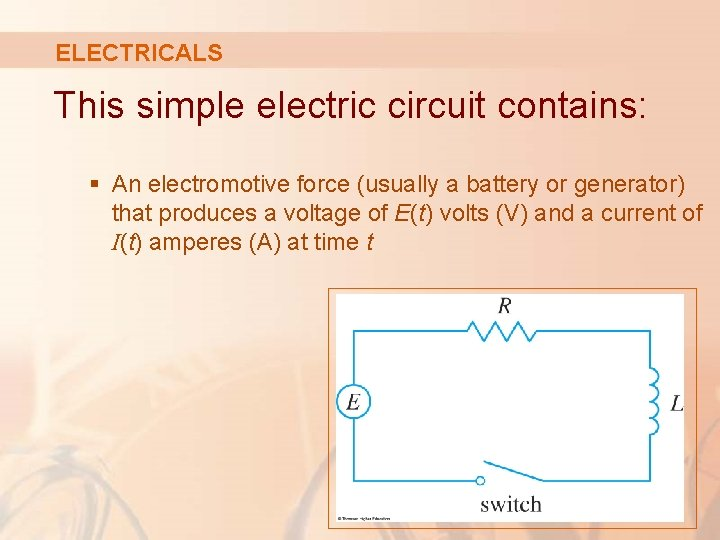ELECTRICALS This simple electric circuit contains: § An electromotive force (usually a battery or generator) that produces a voltage of E(t) volts (V) and a current of I(t) amperes (A) at time t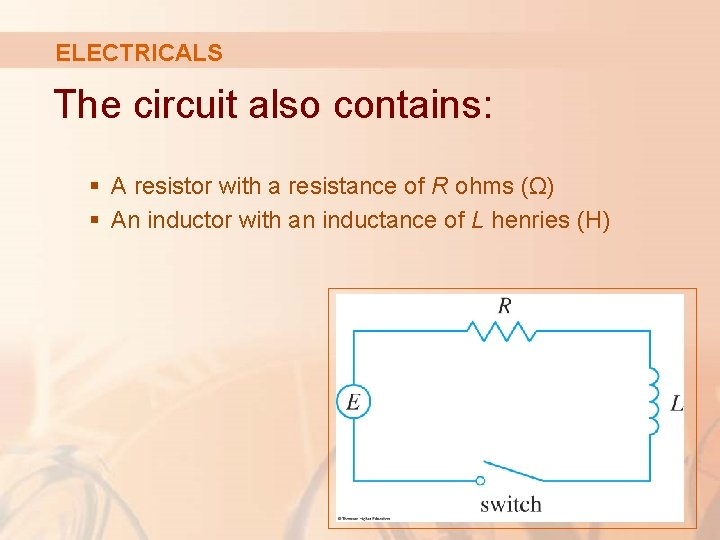ELECTRICALS The circuit also contains: § A resistor with a resistance of R ohms (Ω) § An inductor with an inductance of L henries (H)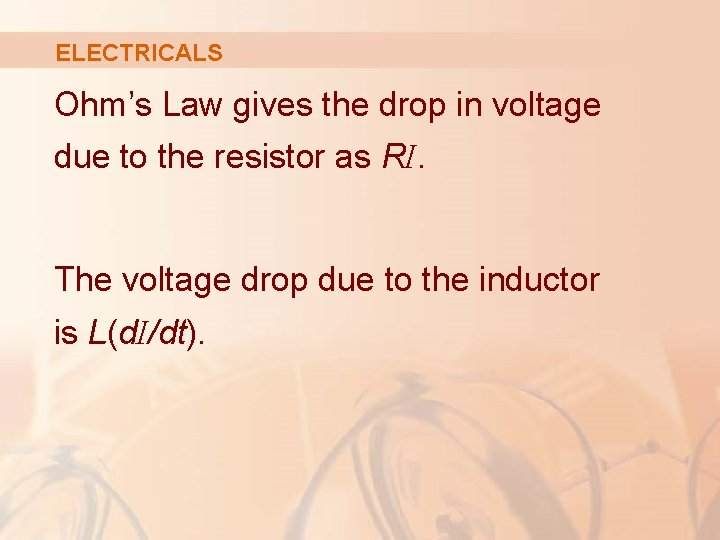ELECTRICALS Ohm’s Law gives the drop in voltage due to the resistor as RI. The voltage drop due to the inductor is L(d. I/dt).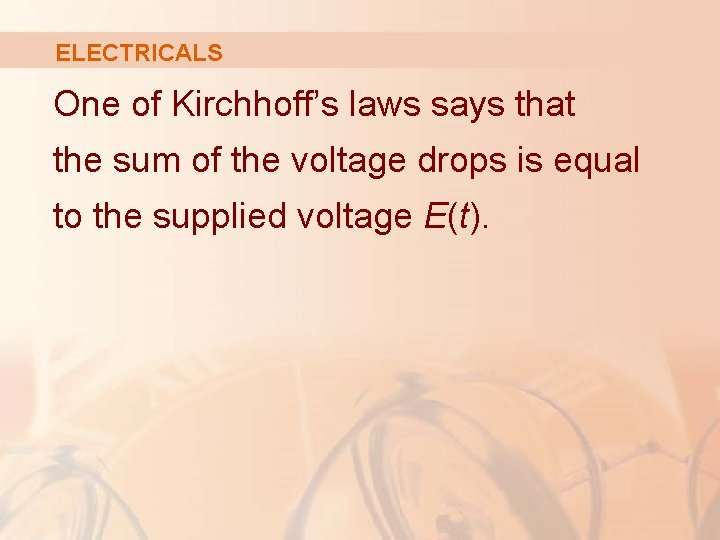ELECTRICALS One of Kirchhoff’s laws says that the sum of the voltage drops is equal to the supplied voltage E(t).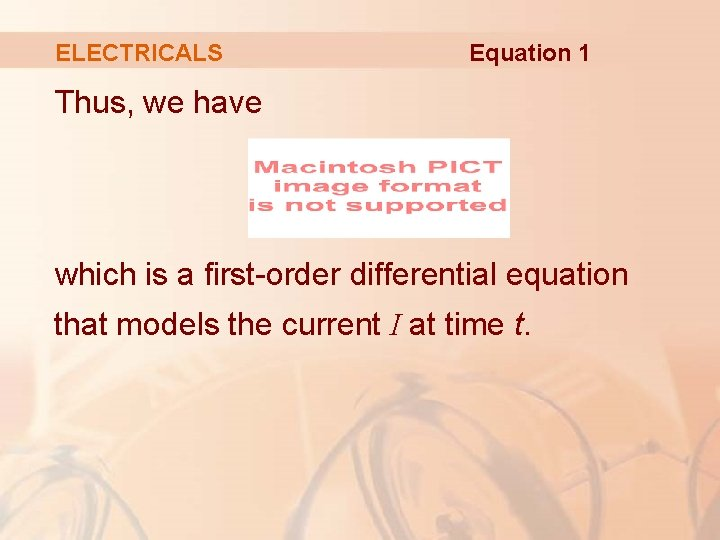ELECTRICALS Equation 1 Thus, we have which is a first-order differential equation that models the current I at time t.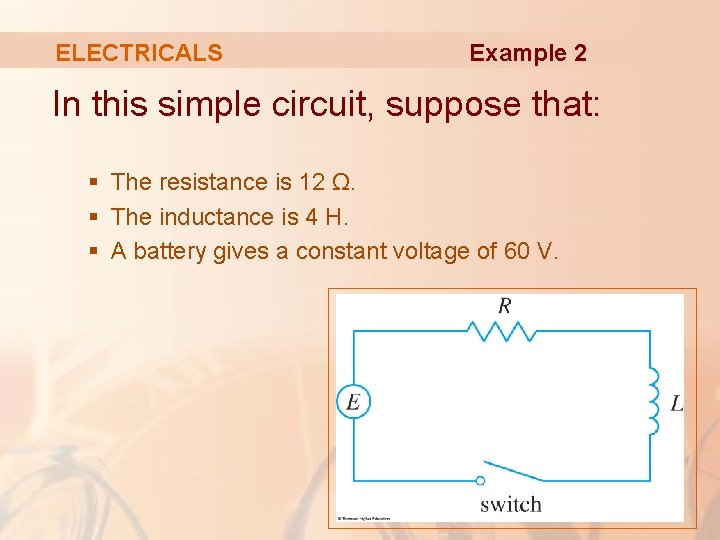ELECTRICALS Example 2 In this simple circuit, suppose that: § The resistance is 12 Ω. § The inductance is 4 H. § A battery gives a constant voltage of 60 V.ELECTRICALS Example 2 a. Identify any equilibrium solutions. b. If the switch is closed when t = 0 so the current starts with I(0) = 0, use the direction field to sketch the solution curve.ELECTRICALS Example 2 c. Draw a direction field for Equation 1 with those values. d. What can you say about the limiting value of the current?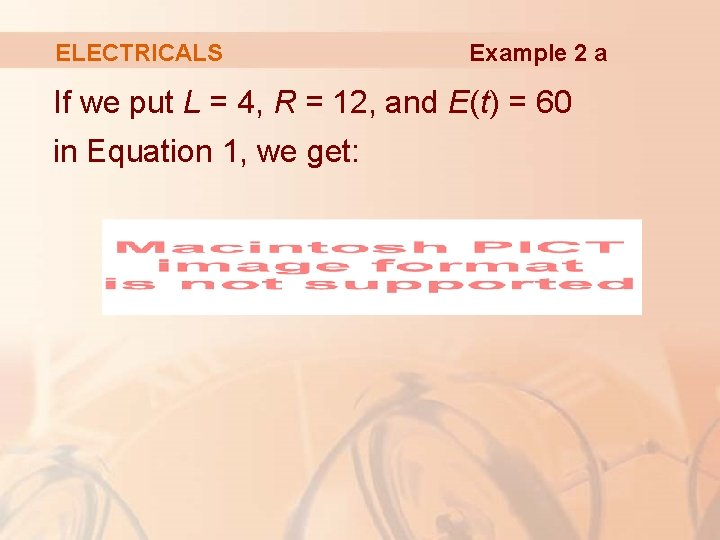ELECTRICALS Example 2 a If we put L = 4, R = 12, and E(t) = 60 in Equation 1, we get: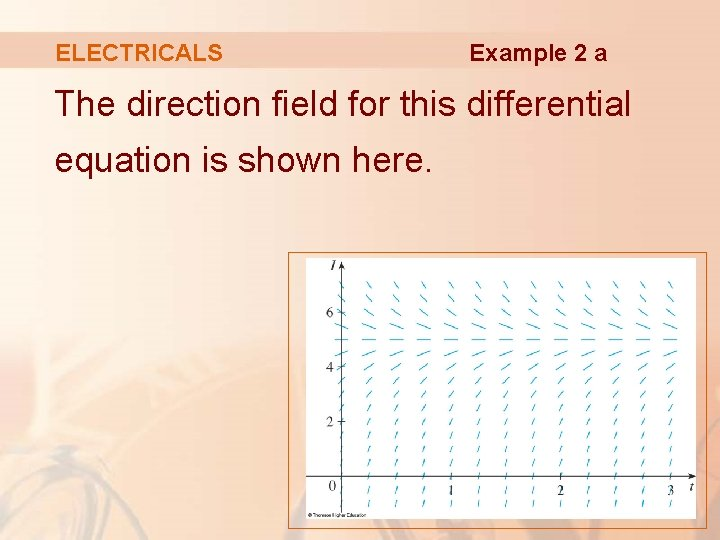ELECTRICALS Example 2 a The direction field for this differential equation is shown here.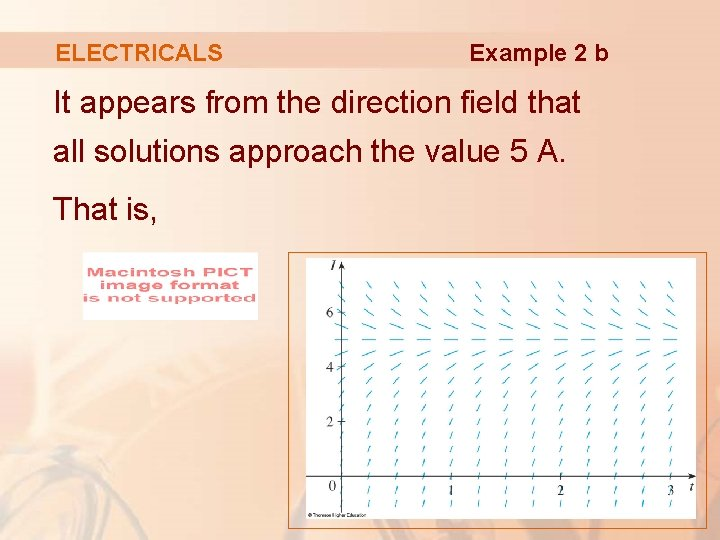ELECTRICALS Example 2 b It appears from the direction field that all solutions approach the value 5 A. That is,ELECTRICALS Example 2 c It appears that the constant function I(t) = 5 is an equilibrium solution. § Indeed, we can verify this directly from the differential equation d. I/dt = 15 – 3 I.ELECTRICALS Example 2 c If I(t) = 5, then § The left side is: d. I/dt = 0 § The right side is: 15 – 3(5) = 0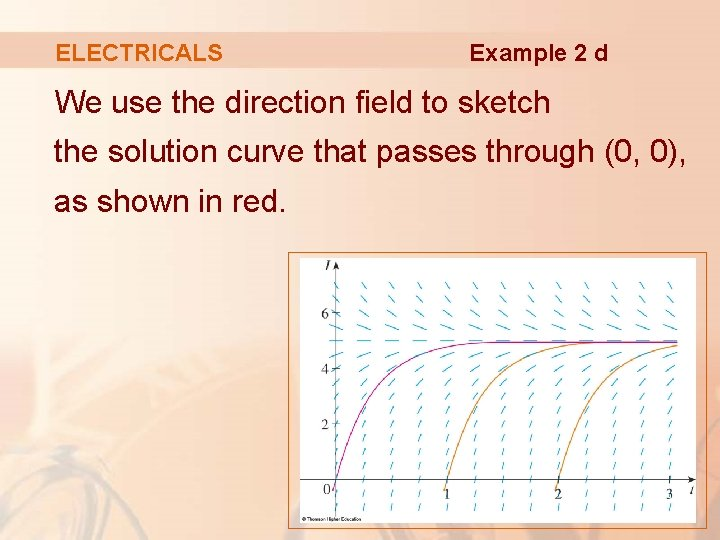ELECTRICALS Example 2 d We use the direction field to sketch the solution curve that passes through (0, 0), as shown in red.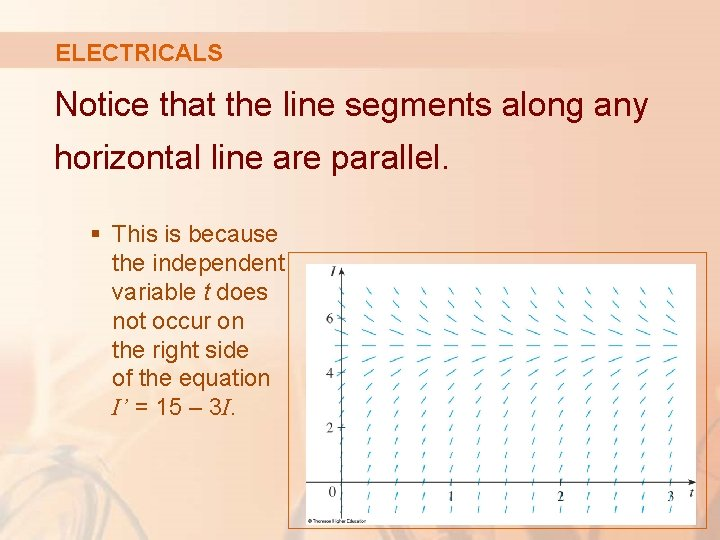ELECTRICALS Notice that the line segments along any horizontal line are parallel. § This is because the independent variable t does not occur on the right side of the equation I’ = 15 – 3 I.AUTONOMOUS DIFFERENTIAL EQUATION In general, a differential equation of the form y’ = f (y) in which the independent variable is missing from the right side, is called autonomous.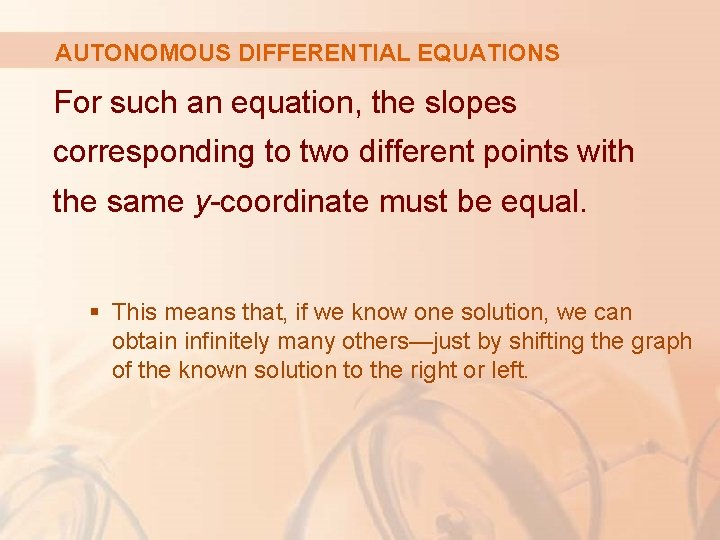AUTONOMOUS DIFFERENTIAL EQUATIONS For such an equation, the slopes corresponding to two different points with the same y-coordinate must be equal. § This means that, if we know one solution, we can obtain infinitely many others—just by shifting the graph of the known solution to the right or left.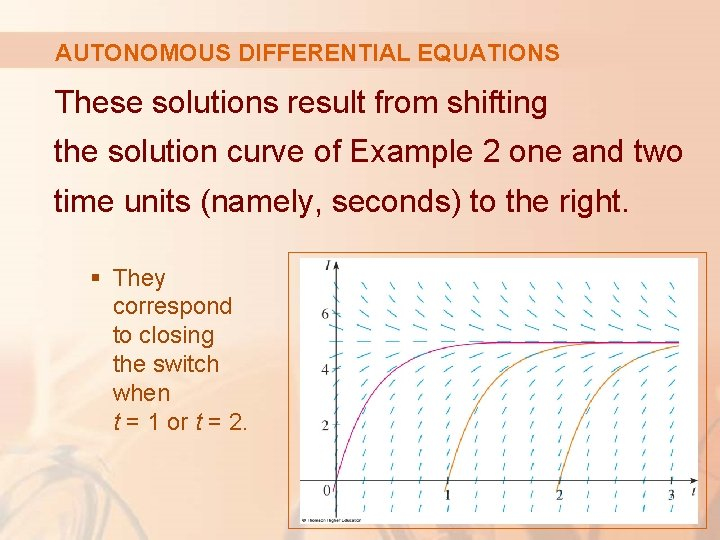AUTONOMOUS DIFFERENTIAL EQUATIONS These solutions result from shifting the solution curve of Example 2 one and two time units (namely, seconds) to the right. § They correspond to closing the switch when t = 1 or t = 2.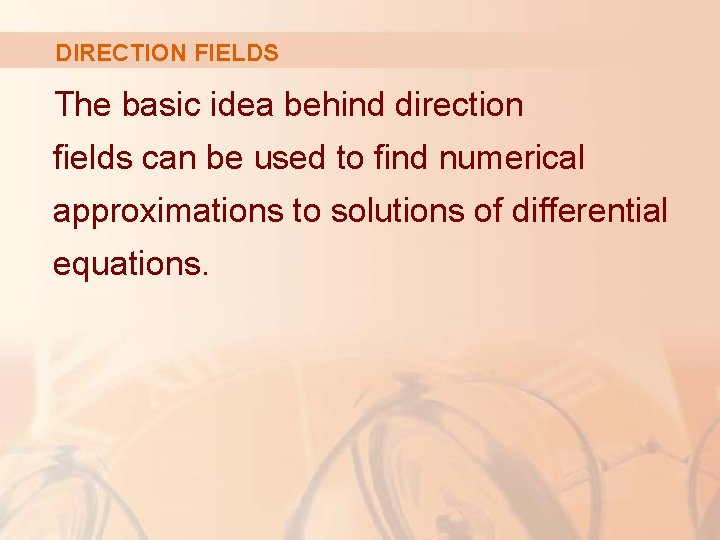DIRECTION FIELDS The basic idea behind direction fields can be used to find numerical approximations to solutions of differential equations.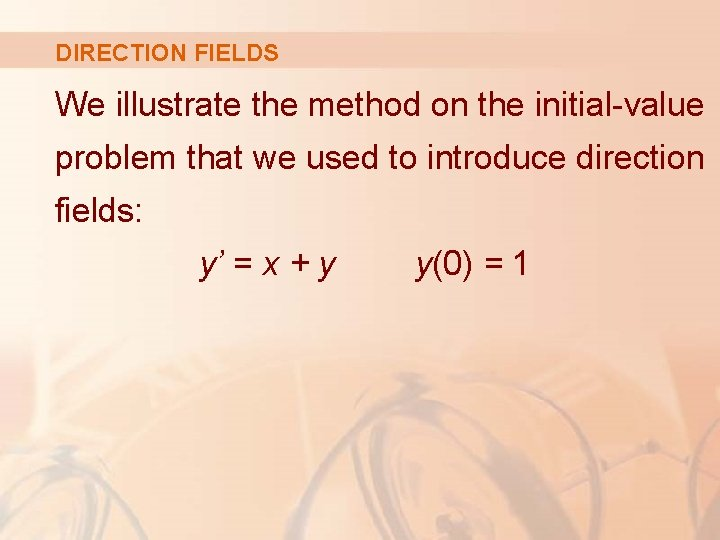DIRECTION FIELDS We illustrate the method on the initial-value problem that we used to introduce direction fields: y’ = x + y y(0) = 1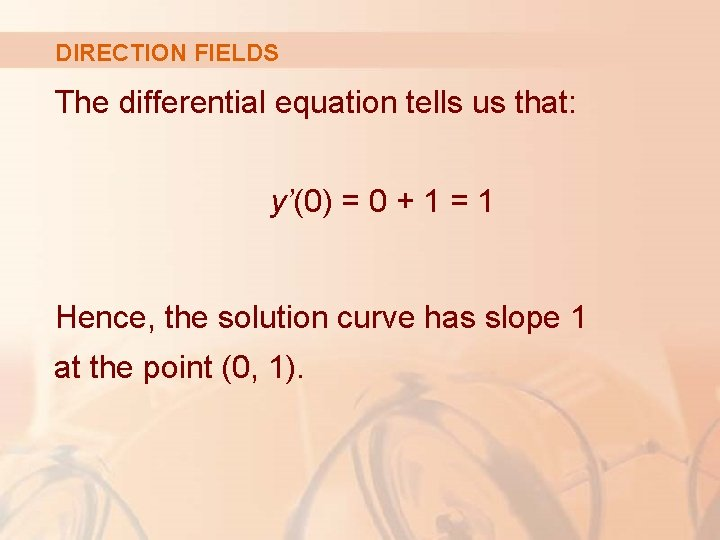DIRECTION FIELDS The differential equation tells us that: y’(0) = 0 + 1 = 1 Hence, the solution curve has slope 1 at the point (0, 1).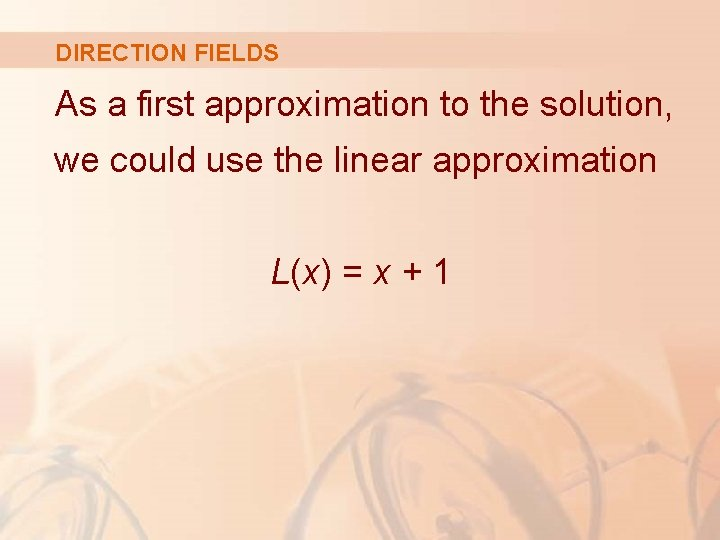DIRECTION FIELDS As a first approximation to the solution, we could use the linear approximation L(x) = x + 1DIRECTION FIELDS In other words, we could use the tangent line at (0, 1) as a rough approximation to the solution curve.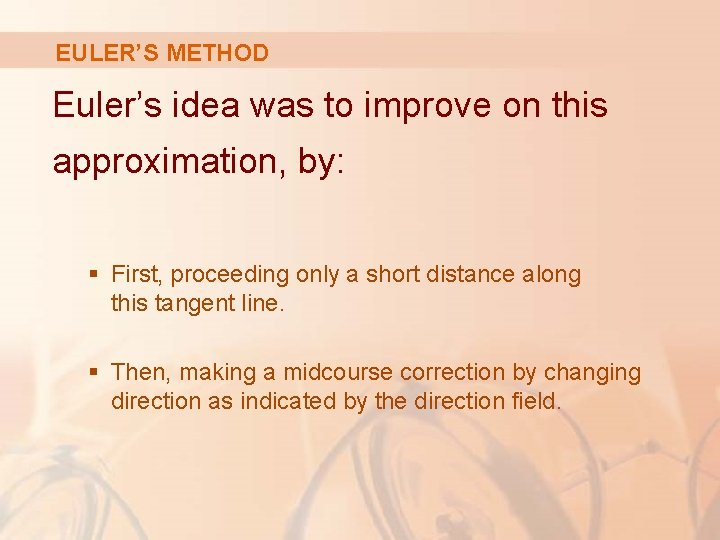EULER’S METHOD Euler’s idea was to improve on this approximation, by: § First, proceeding only a short distance along this tangent line. § Then, making a midcourse correction by changing direction as indicated by the direction field.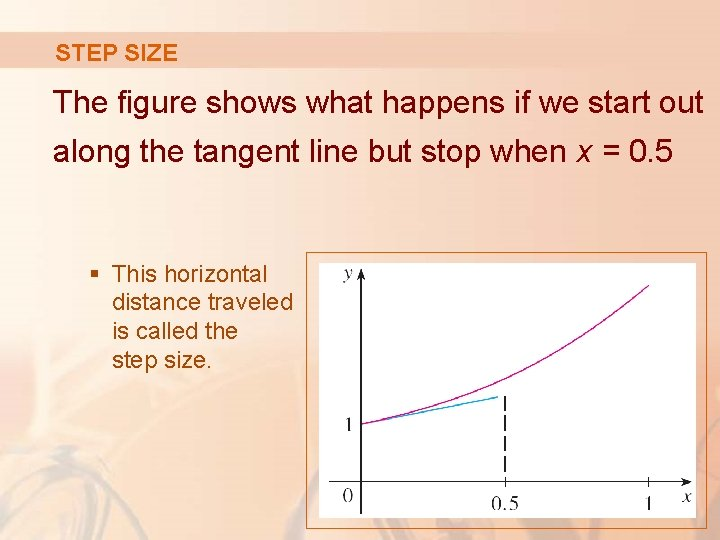STEP SIZE The figure shows what happens if we start out along the tangent line but stop when x = 0. 5 § This horizontal distance traveled is called the step size.EULER’S METHOD Since L(0. 5) = 1. 5, we have y(0. 5) ≈ 1. 5 and we take (0. 5, 1. 5) as the starting point for a new line segment.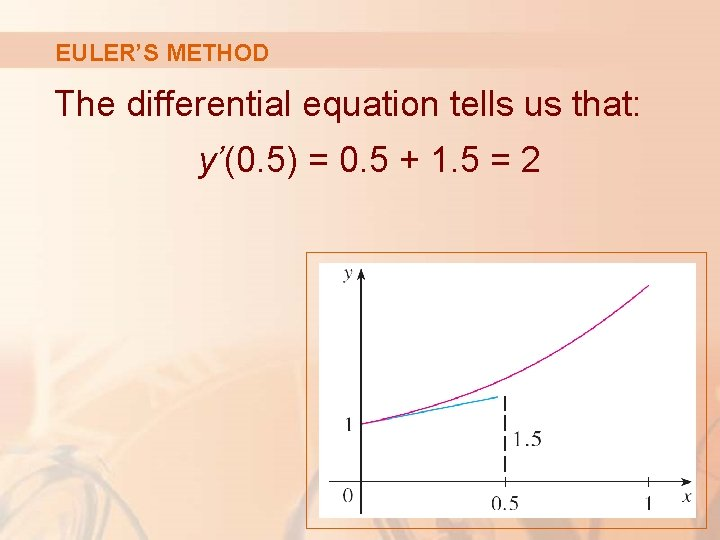EULER’S METHOD The differential equation tells us that: y’(0. 5) = 0. 5 + 1. 5 = 2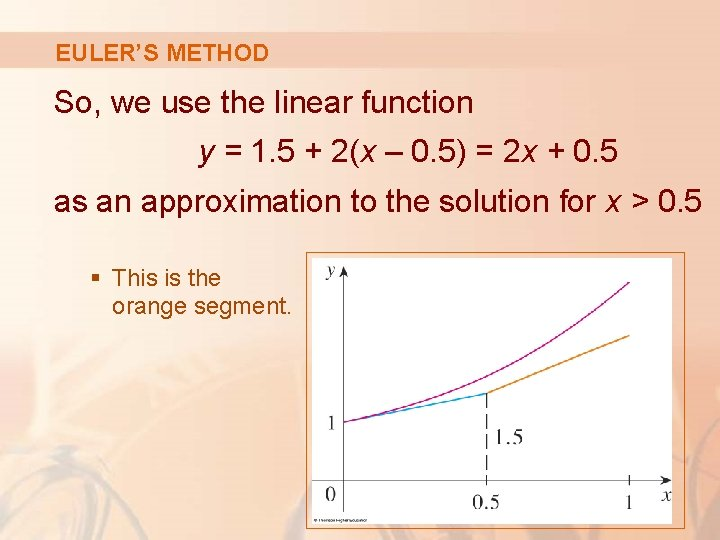EULER’S METHOD So, we use the linear function y = 1. 5 + 2(x – 0. 5) = 2 x + 0. 5 as an approximation to the solution for x > 0. 5 § This is the orange segment.EULER’S METHOD If we decrease the step size from 0. 5 to 0. 25, we get the better Euler approximation shown here.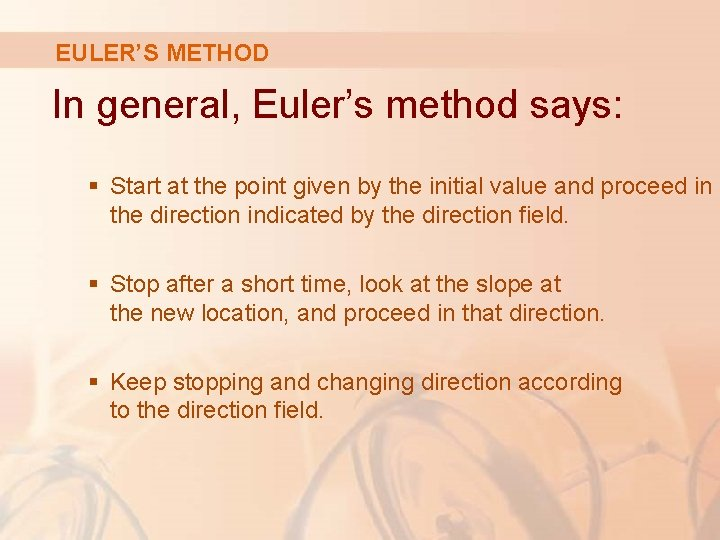EULER’S METHOD In general, Euler’s method says: § Start at the point given by the initial value and proceed in the direction indicated by the direction field. § Stop after a short time, look at the slope at the new location, and proceed in that direction. § Keep stopping and changing direction according to the direction field.EULER’S METHOD Euler’s method does not produce the exact solution to an initial-value problem. It gives approximations.EULER’S METHOD However, by decreasing the step size (thus increasing the number of midcourse corrections), we obtain successively better approximations to the exact solution.EULER’S METHOD We understand this when we compare three figures.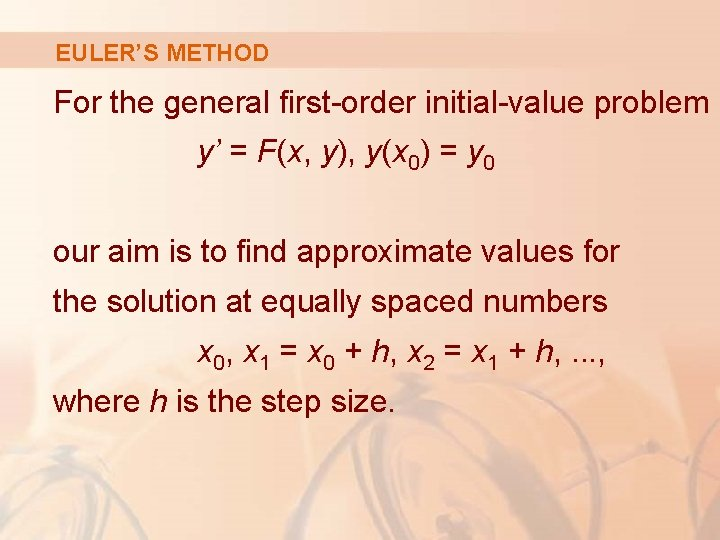EULER’S METHOD For the general first-order initial-value problem y’ = F(x, y), y(x 0) = y 0 our aim is to find approximate values for the solution at equally spaced numbers x 0, x 1 = x 0 + h, x 2 = x 1 + h, . . . , where h is the step size.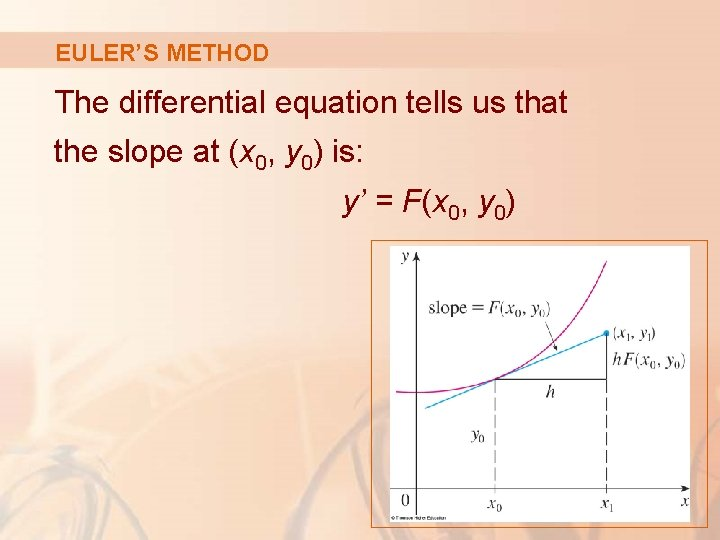EULER’S METHOD The differential equation tells us that the slope at (x 0, y 0) is: y’ = F(x 0, y 0)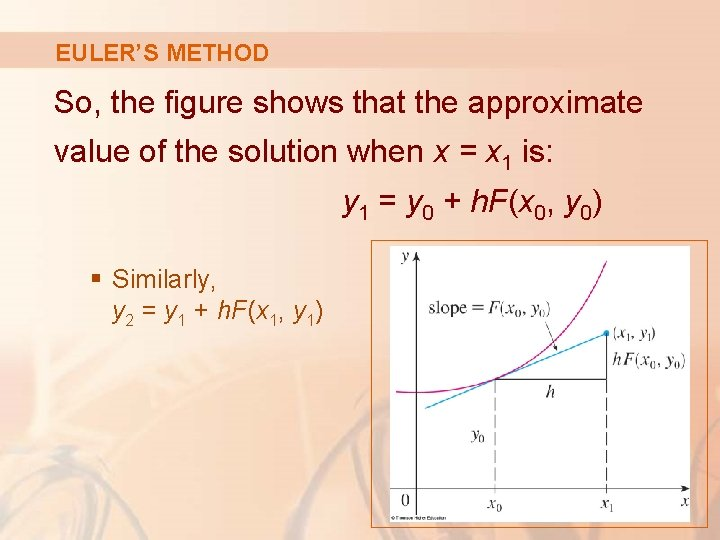EULER’S METHOD So, the figure shows that the approximate value of the solution when x = x 1 is: y 1 = y 0 + h. F(x 0, y 0) § Similarly, y 2 = y 1 + h. F(x 1, y 1)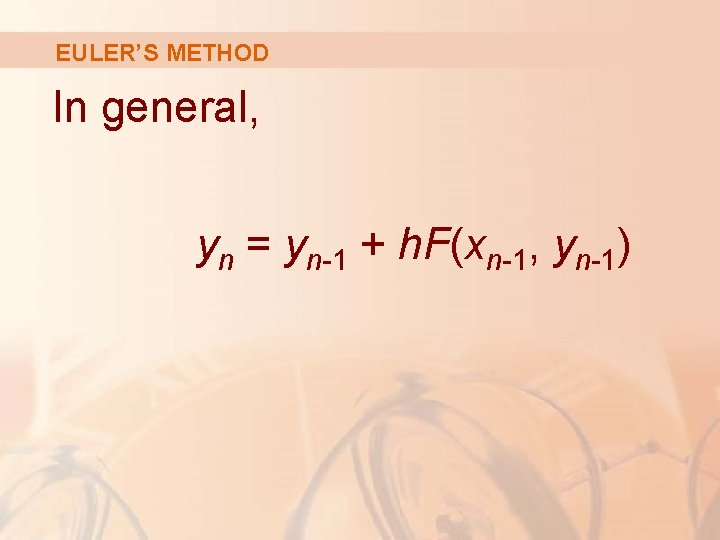EULER’S METHOD In general, yn = yn-1 + h. F(xn-1, yn-1)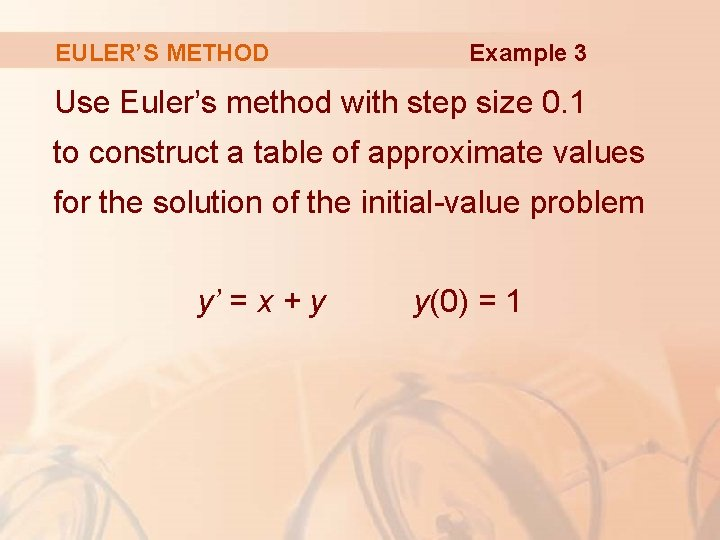EULER’S METHOD Example 3 Use Euler’s method with step size 0. 1 to construct a table of approximate values for the solution of the initial-value problem y’ = x + y y(0) = 1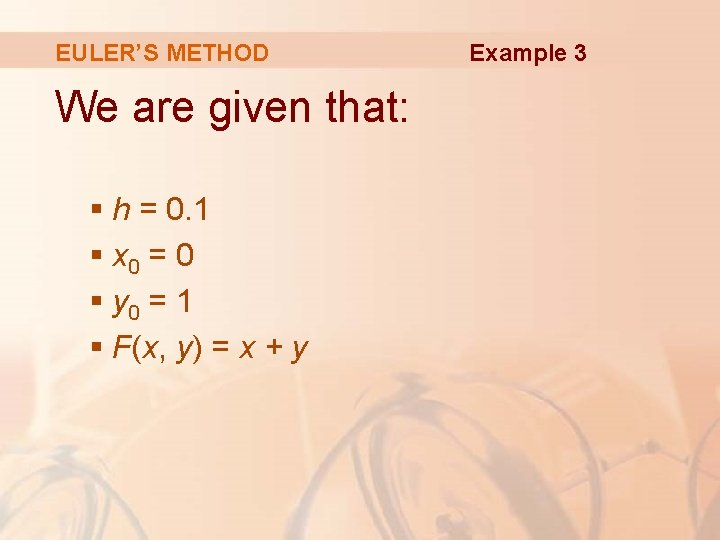EULER’S METHOD We are given that: § h = 0. 1 § x 0 = 0 § y 0 = 1 § F(x, y) = x + y Example 3EULER’S METHOD Example 3 Thus, we have: § y 1 = y 0 + h F(x 0, y 0) = 1 + 0. 1(0 + 1) = 1. 1 § y 2 = y 1 + h F(x 1, y 1) = 1. 1 + 0. 1(0. 1 + 1. 1) = 1. 22 § y 3 = y 2 + h F(x 2, y 2) = 1. 22 + 0. 1(0. 2 + 1. 22) = 1. 362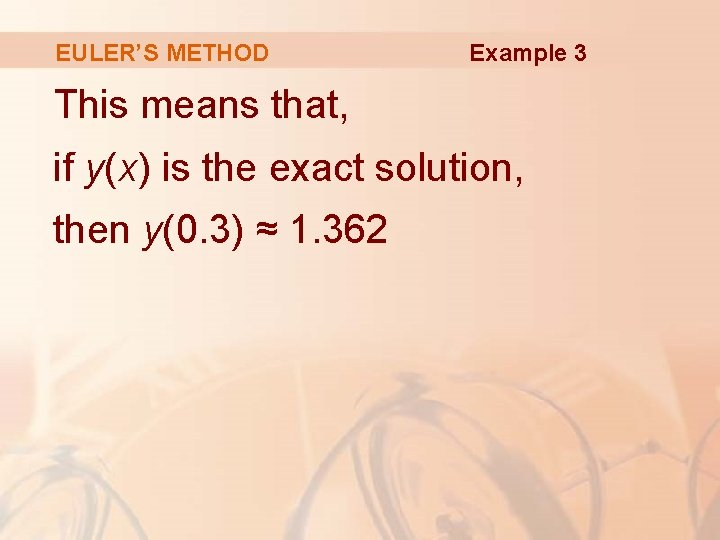EULER’S METHOD Example 3 This means that, if y(x) is the exact solution, then y(0. 3) ≈ 1. 362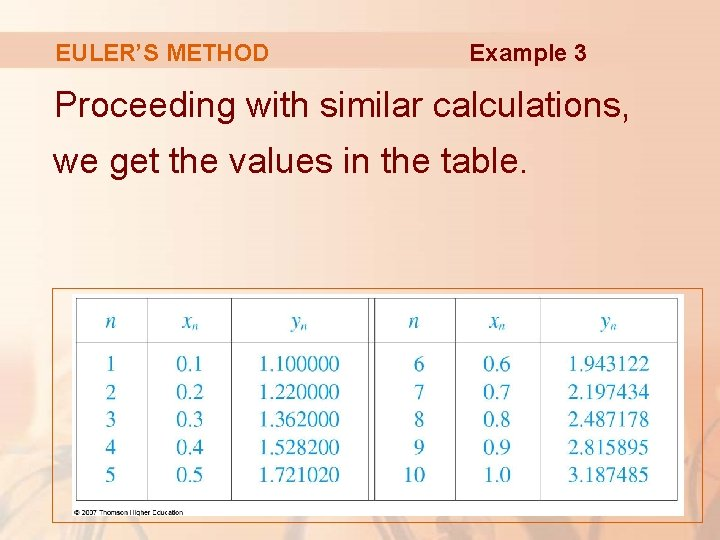EULER’S METHOD Example 3 Proceeding with similar calculations, we get the values in the table.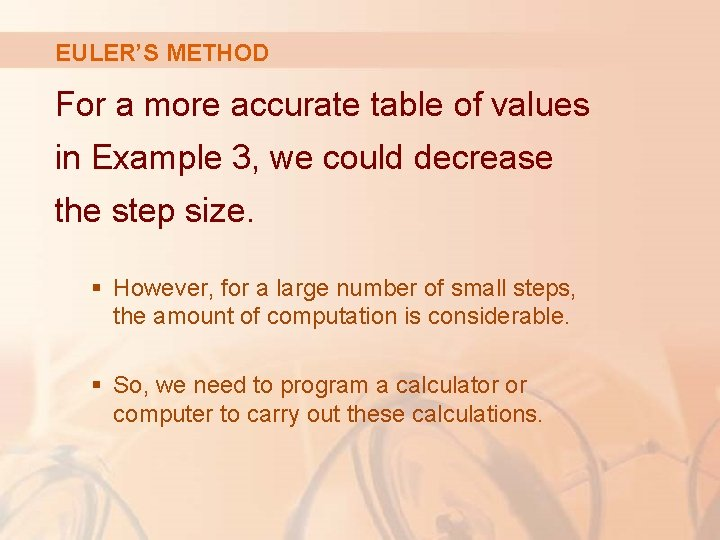EULER’S METHOD For a more accurate table of values in Example 3, we could decrease the step size. § However, for a large number of small steps, the amount of computation is considerable. § So, we need to program a calculator or computer to carry out these calculations.EULER’S METHOD This table shows the results of applying Euler’s method with decreasing step size to the initial-value problem of Example 3.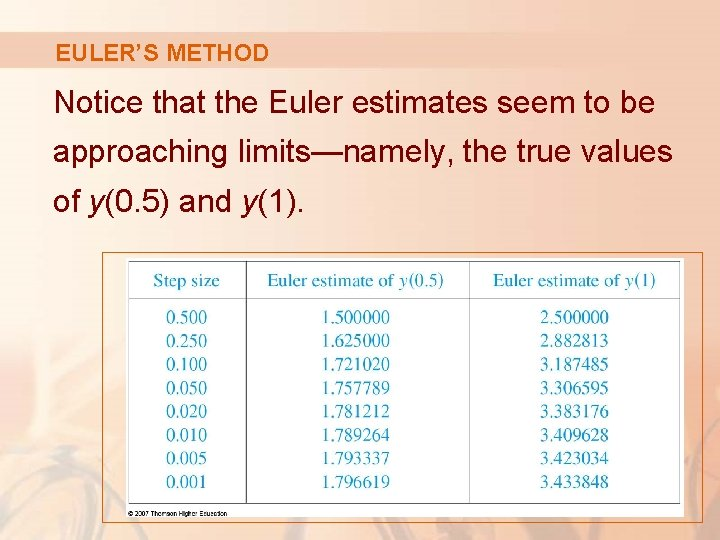EULER’S METHOD Notice that the Euler estimates seem to be approaching limits—namely, the true values of y(0. 5) and y(1).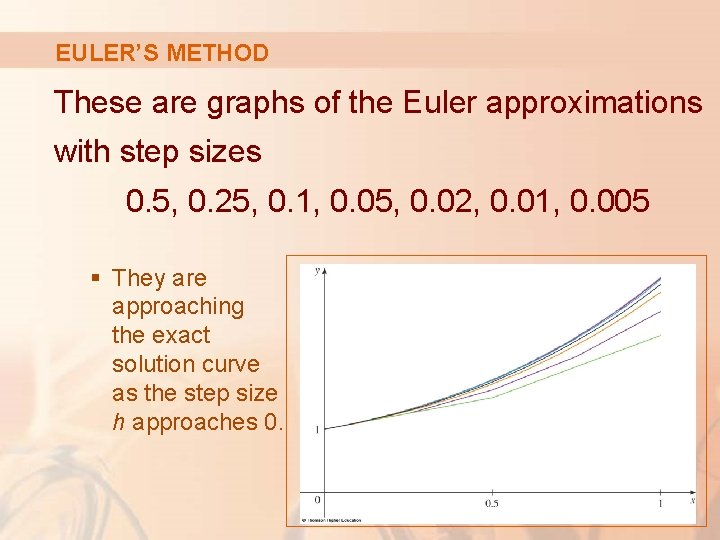EULER’S METHOD These are graphs of the Euler approximations with step sizes 0. 5, 0. 25, 0. 1, 0. 05, 0. 02, 0. 01, 0. 005 § They are approaching the exact solution curve as the step size h approaches 0.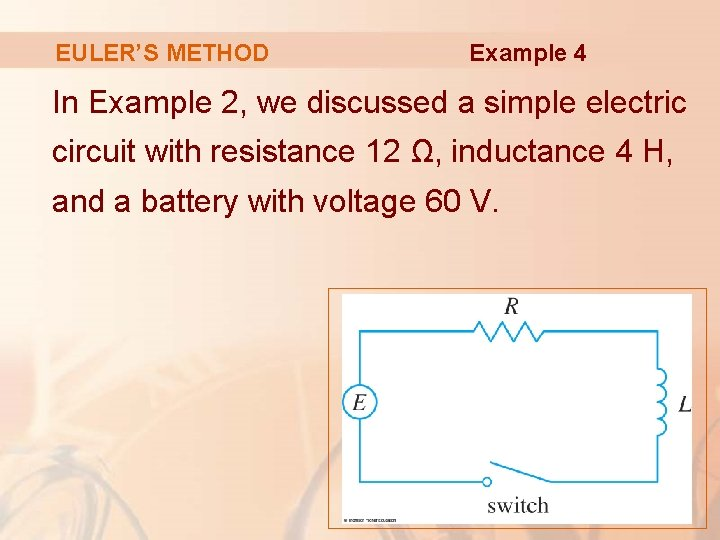EULER’S METHOD Example 4 In Example 2, we discussed a simple electric circuit with resistance 12 Ω, inductance 4 H, and a battery with voltage 60 V.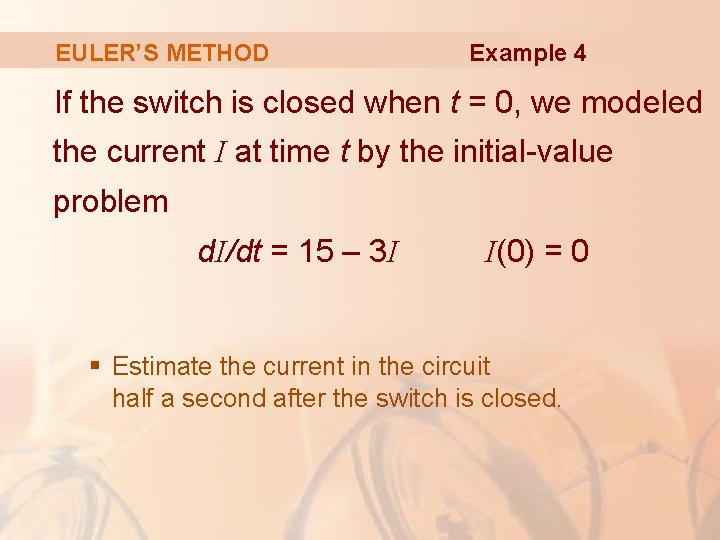EULER’S METHOD Example 4 If the switch is closed when t = 0, we modeled the current I at time t by the initial-value problem d. I/dt = 15 – 3 I I(0) = 0 § Estimate the current in the circuit half a second after the switch is closed.EULER’S METHOD Example 4 We use Euler’s method with: § § F(t, I) = 15 – 3 I t 0 = 0 I 0 = 0 Step size h = 0. 1 second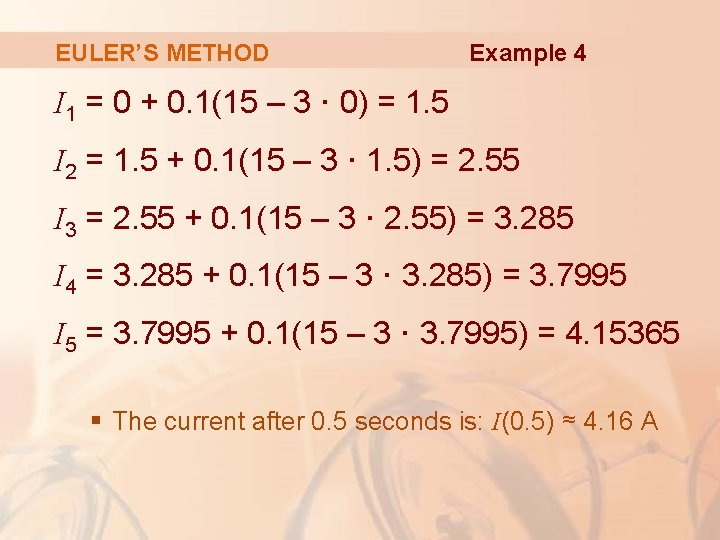EULER’S METHOD Example 4 I 1 = 0 + 0. 1(15 – 3 · 0) = 1. 5 I 2 = 1. 5 + 0. 1(15 – 3 · 1. 5) = 2. 55 I 3 = 2. 55 + 0. 1(15 – 3 · 2. 55) = 3. 285 I 4 = 3. 285 + 0. 1(15 – 3 · 3. 285) = 3. 7995 I 5 = 3. 7995 + 0. 1(15 – 3 · 3. 7995) = 4. 15365 § The current after 0. 5 seconds is: I(0. 5) ≈ 4. 16 A